# How to Calculate and Solve for Petrosky-Farshad Gas Solubility Parameter | The Calculator Encyclopedia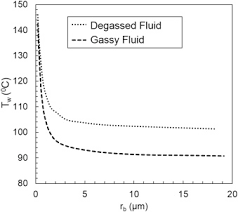The image above represents petrosky-farshad gas solubility parameter.

To compute for the petrosky-farshad gas solubility parameter, two essential parameters are needed and these parameters are API Gravity (°API) and temperature (°Rankine) (T).

The formula for calculating the petrosky-farshad gas solubility parameter:

Rs = [7.716(10-4) (°API)1.541] – [4.561(10-5) (T -460)1.3911]

where:

Rs = Petrosky-Farshad Gas Solubility Parameter, x
°API = API Gravity
T = Temperature (°Rankine)

Let’s solve an example;
Find the petrosky-farshad gas solubility parameter with an API Gravity of 11 and a temperature of 90.

This implies that;

°API = API Gravity = 11
T = Temperature (°Rankine) = 90

Rs = [7.716(10-4) (°API)1.541] – [4.561(10-5) (T -460)1.3911]
Rs = [7.716(10-4) (11)1.541] – [4.561(10-5) (90 – 460)1.3911]
Rs = [7.716(10-4) (11)1.541] – [4.561(10-5) (-370)1.3911]
Rs = [7.716(10-4) (11)1.541] – [4.561(10-5) (NaN)]
Rs = [7.716(10-4) (40.2518)] – [4.561(10-5) (NaN)]
Rs = [0.03105] – [4.561(10-5) (NaN)]
Rs = 0.03105 – NaN
Rs = NaN

Therefore, the Petrosky-Farshad gas solubility parameter, x is NaN.

Nickzom Calculator – The Calculator Encyclopedia is capable of calculating the petrosky-farshad gas solubility parameter.

To get the answer and workings of the petrosky-farshad gas solubility parameter using the Nickzom Calculator – The Calculator Encyclopedia. First, you need to obtain the app.

You can get this app via any of these means:

To get access to the professional version via web, you need to register and subscribe for NGN 1,500 per annum to have utter access to all functionalities.
You can also try the demo version via https://www.nickzom.org/calculator

Apple (Paid) – https://itunes.apple.com/us/app/nickzom-calculator/id1331162702?mt=8
Once, you have obtained the calculator encyclopedia app, proceed to the Calculator Map, then click on Petroleum under EngineeringNow, Click on Fluid Properties under Petroleum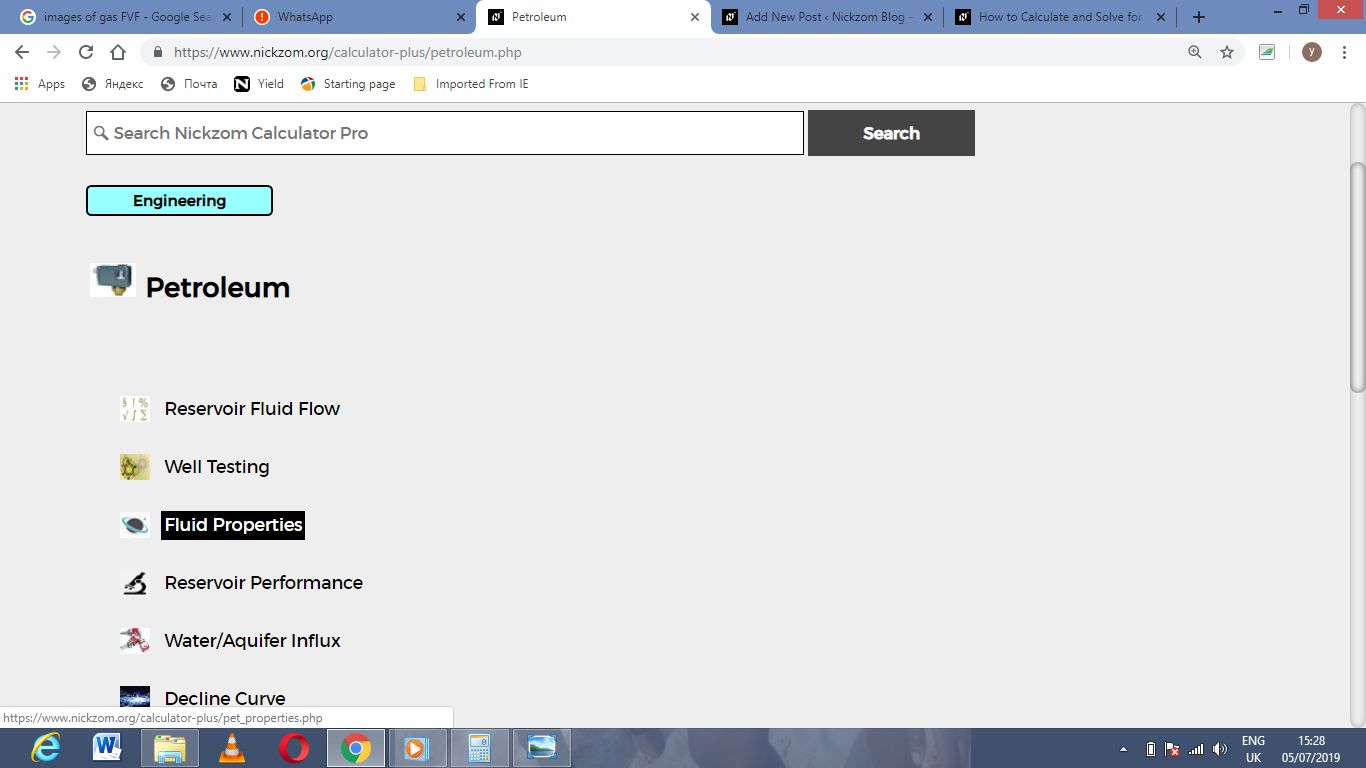Now, Click on Petrosky-Farshad Gas Solubility Parameter under Fluid PropertiesThe screenshot below displays the page or activity to enter your values, to get the answer for the petroksy-farshad gas solubility parameter according to the respective parameters which are the API Gravity (°API) and temperature (°Rankine) (T).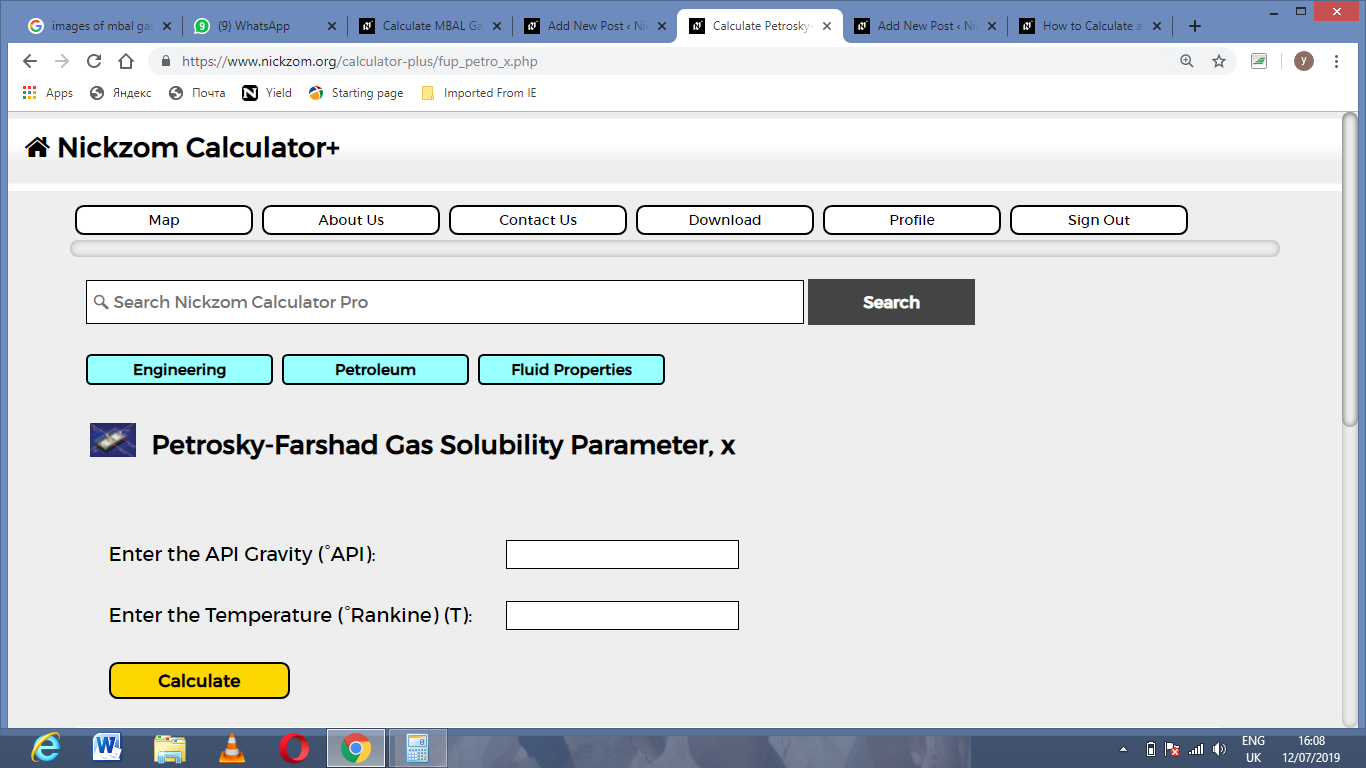Now, enter the values appropriately and accordingly for the parameters as required by the API Gravity (°API) is 11 and temperature (°Rankine) (T) is 90.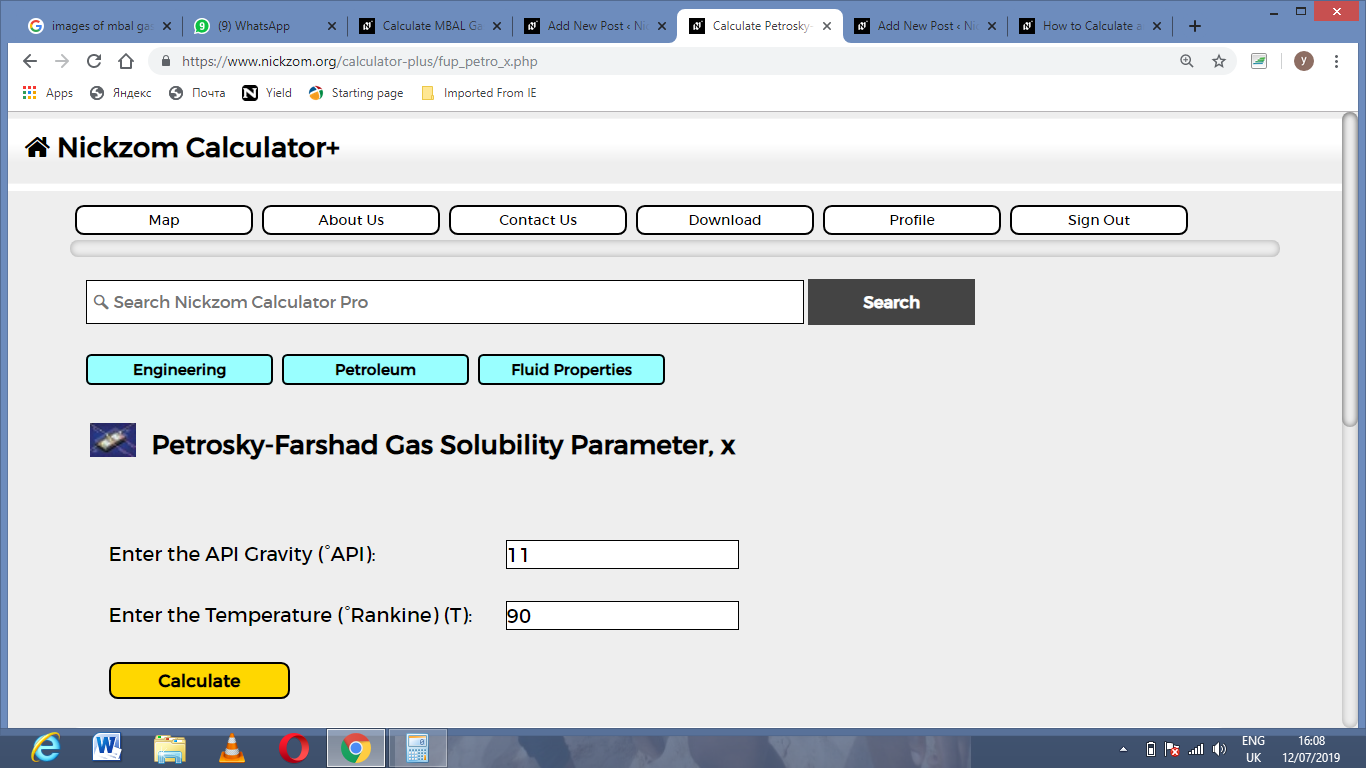Finally, Click on Calculate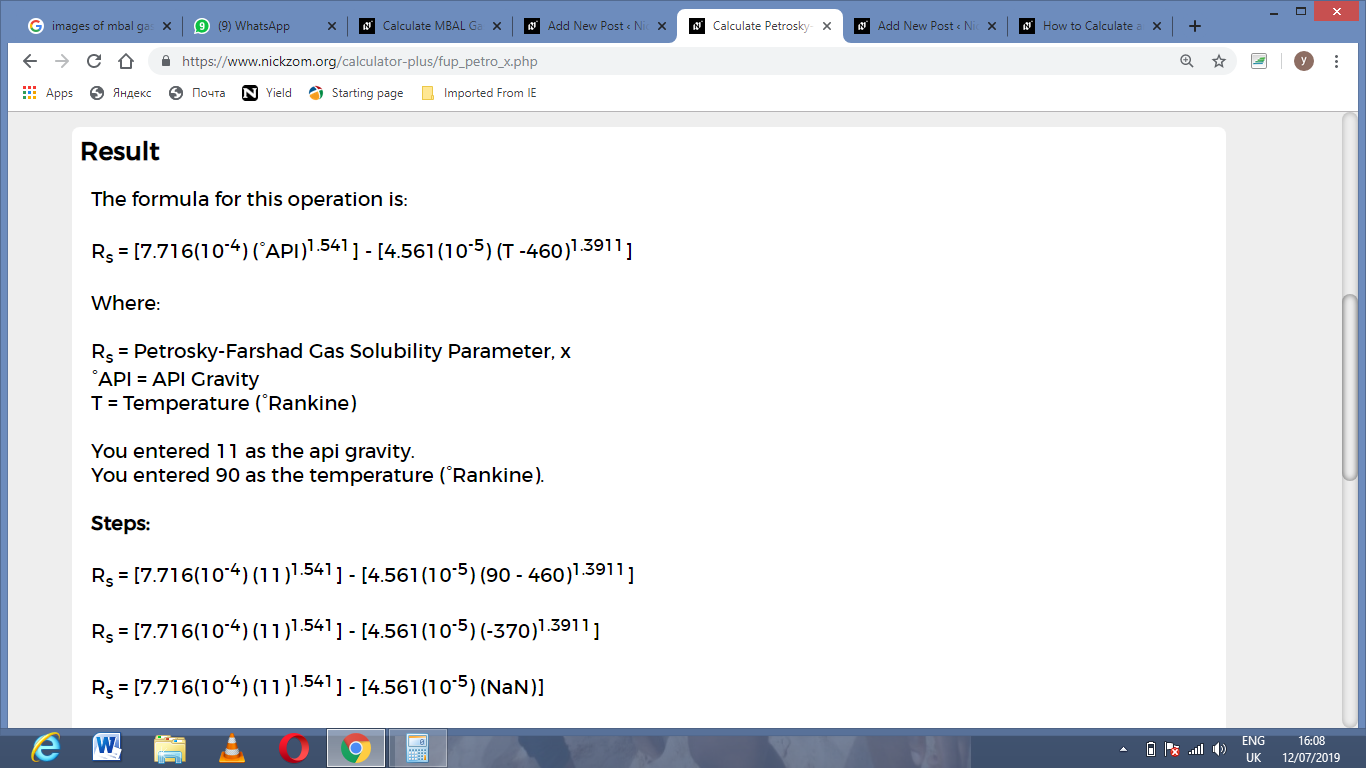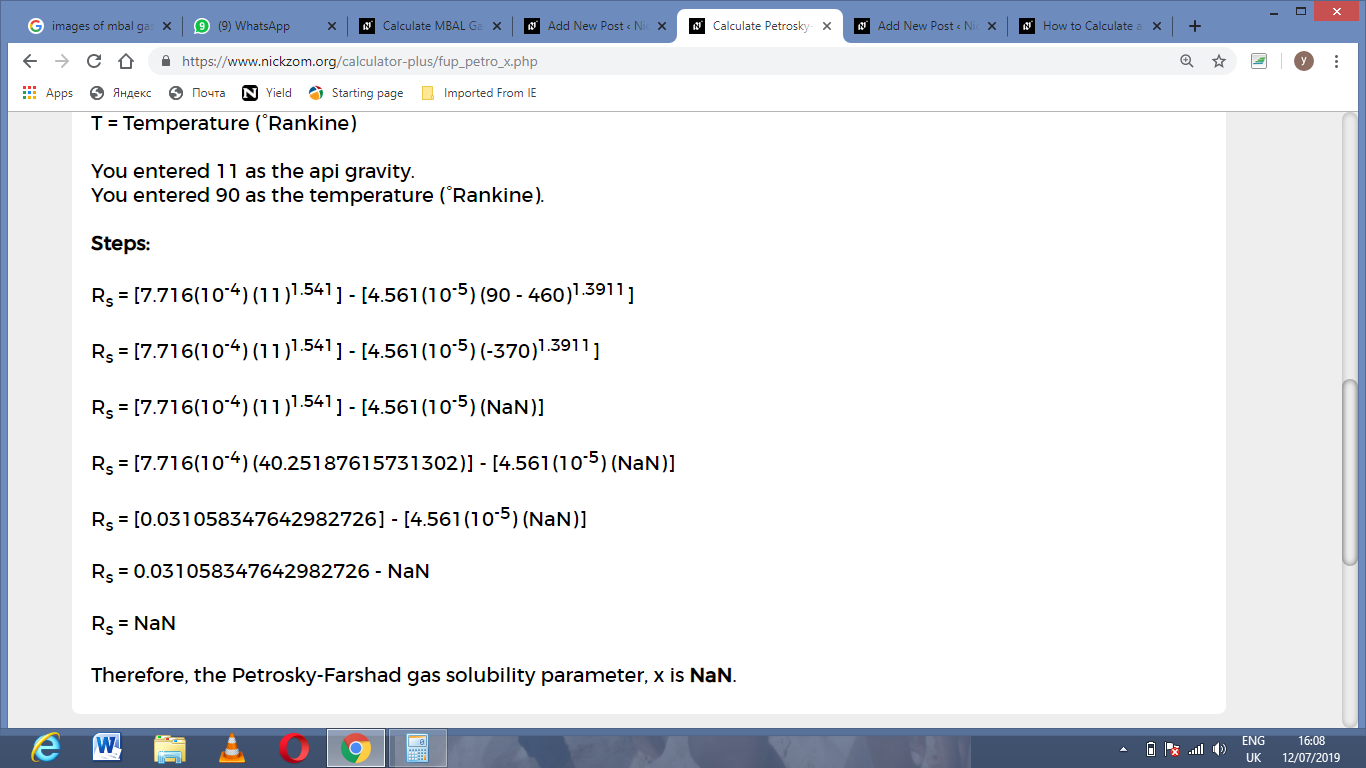As you can see from the screenshot above, Nickzom Calculator– The Calculator Encyclopedia solves for the petrosky-farshad gas solubility parameter and presents the formula, workings and steps too.